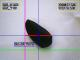border points
Blaz from Slovenia  [6 posts]
13 years
 Hi! I have a little problem... I am trying to find min_x and max_x value on a speciefied Y (for example COG_Y). I uploaded a picture where is drawn a red line between two points which are the global max_x and global min_x. I would like to know the lokal max_x and lokal min_x. In other words max_x and min_x on y=COG_Y and draw the line between them. Any suggestions? bye BlazAnonymous 13 years
 Blaz, Yes, you can use the edge probe to look along the Y line for the edges of the object. See the robofile below for a quick example using a test image. Note that the trick is to use the [variable] expression in the edge probe GUI instead of a static number. This allows for dynamic configuration of GUI interfaces. STeven.program.roboBlaz from Slovenia  [6 posts] 13 years
 Thanks STeven! Edge probe is the right thing. But I have an another problem... When you do the Edge Probe an you find two edges points. The position xy (of the points) is writen in an array PROBED_EDGES. I would like to use this values in some equations. I tried this way, but is not the right way because I can not get the variables I need. Dim point ReDim point(2,2) point(2,2) = GetArrayVariable("PROBED_EDGES") x1=point(0,0) y1=point(0,1) x2=point(1,0) y2=point(1,1) SetVariable "Y1", y1 SetVariable "Y2", y2 The resoult is 0 for both... Any Ideas? Thank you in advance BlazBlaz from Slovenia  [6 posts] 13 years
 Just a correction: The result is not 0 is nothing (is empty)! BlazAnonymous 13 years
 Blaz, In your case try points = GetArrayVariable("PROBED_EDGES") x1=point(0) y1=point(1) x2=point(2) y2=point(3) SetVariable "Y1", y1 SetVariable "Y2", y2 or if you plan to use those values in other modules where typically one types in just a number for a shorthand try [PROBED_EDGES:0] for the first element [PROBED_EDGES:1] for the second, etc. instead of the number that you would normally type in. The [] tell the module that it is a variable and an expression to be evalulated before the actual number is used. So you can also use [PROBED_EDGES:0+5] if you'd like. STeven.Blaz from Slovenia  [6 posts] 13 years
 Thank you STeven!!! I have tried this way before, but I made a stupid mistake.... I wrote points (with s) = GetArrayVariable("PROBED_EDGES") and then x1 = point (without s) (0) .... And then I tought that is a problem of dimensions, etc.. OK Thank you for everthing! bye Blaz

 This forum thread has been closed due to inactivity (more than 4 months) or number of replies (more than 50 messages). Please start a New Post and enter a new forum thread with the appropriate title.